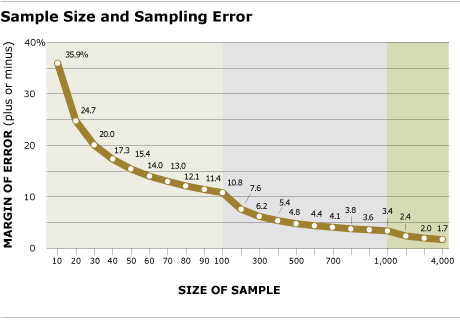Probability sampling, where a small randomly selected sample of the population can be used to estimate the distribution of an attitude or opinion in the entire population with statistical confidence, had traditionally provided the foundation for survey research and political polling. The basis of probability-based random sampling is that every member of the population must have a known, non-zero chance of being selected. Probability sampling provides the means by which the margin of sampling error can be calculated and the level of confidence in survey estimates reported. Sampling error results from collecting data from some rather than all members of the population and is highly dependent on the size of the sample.We report a margin of sampling error for the total sample for each survey and sometimes for key subgroups (e.g., registered voters, Democrats, Republicans, etc.). For example, the sampling error for a typical Pew Research Center national survey of 1,500 completed interviews is plus or minus 3 percentage points with a 95% confidence interval. This means that in 95 out of every 100 samples of the same size and type, the results we would obtain will vary by no more than plus or minus 3 percentage points from the result we would get if we could interview every member of the population. Thus, the chances are very high (95 out of 100) that any sample we draw will be within 3 points of the true population value. The margins of sampling error we report take into account the effect of weighting.

### Nonprobability-based sampling

For some surveys, it may not be feasible or practical to draw a random probability-based sample of the population. In these cases, various types of nonprobability-based samples may be used, such as convenience, volunteer, purposive, and quota samples. Nonprobability samples are often used for qualitative or exploratory research, such as focus groups or in-depth interviews. Many internet panel surveys rely on volunteer samples. Some internet panels, however, have been developed to randomly recruit people using another mode so that random probability sampling methods can be used. For nonprobability-based samples, the relationship between the sample and the population is unknown. That means there is no theoretical basis for computing or reporting a margin of sampling error and thus for knowing how representative the sample is of the population as a whole.